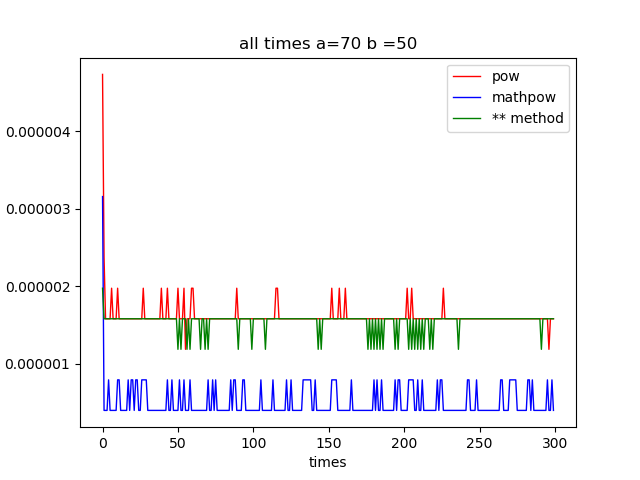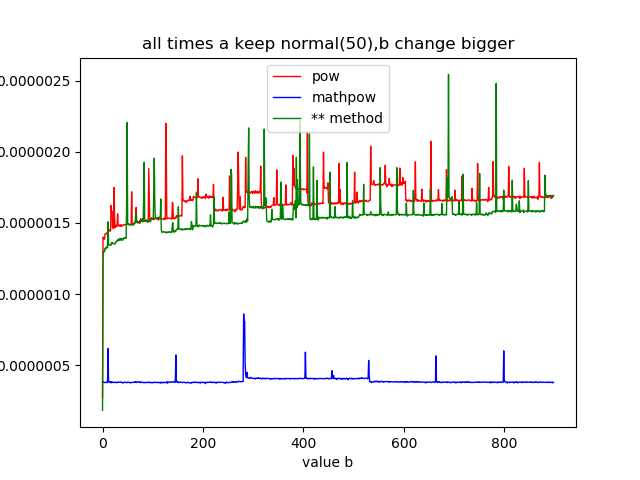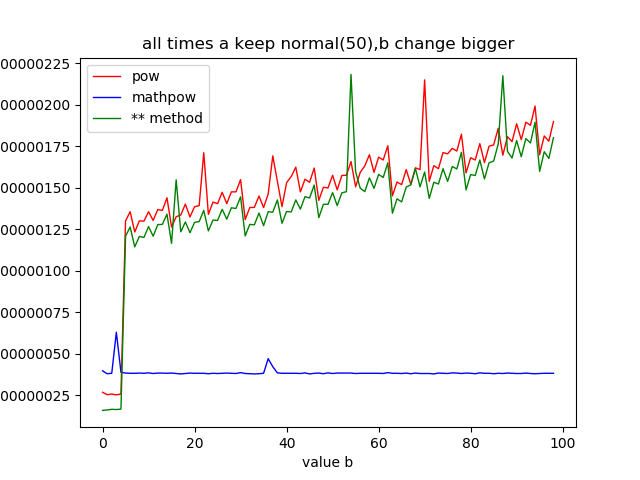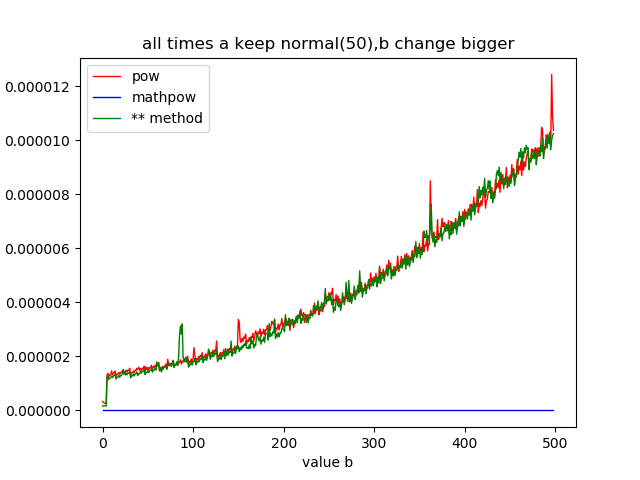## python求次方的三种方式

``````pow(3,4) # 函数用法：pow(x,y[,z])
``````
``import mathmath.pow(3,4)``
``````3**4
``````

## 三种方式的区别

• 使用方式差异
pow支持在次方后，%除一个值
``pow(...)    pow(x, y[, z]) -> number     With two arguments, equivalent to x**y.  With three arguments,    equivalent to (x**y) % z, but may be more efficient (e.g. for longs).``
• 返回值差异
math.pow()返回的值，总是为float，而**和pow()，根据传入的数据类型，返回的值的类型不同，比如pow(2，2)和pow(2,2.0)，前者返回的是int型，后者返回的是float型。

• 上限差异
math.pow()的上线，相比与pow和**两种用法，传入的参数限制得很小。

• 耗时差异
多次带值测试得如下现象（a,b均为int型）：

1，a，b均固定时，重复多次得到每次计算所需要的时间（考虑均值和方差），特征如下：a = 70 and b = 50 for example

• 2，各自，保持b不变，a逐渐增大，如下图；a from 1 to 900 and b keep 50 for example

• 3，各自，保持a不变，b逐渐增大，如下图(未超出math.pow的上限)；b form 1 to 100 and a keep 50 for example ，math.pow not happen error

4，超出math.pow上限后，另外两个图的耗时走势，如下图；a keep 50 and b change from 1 to 500 and set mathpow =0

## 总结

math.pow的优缺点是明显的，即，上限小，但运算速度快。